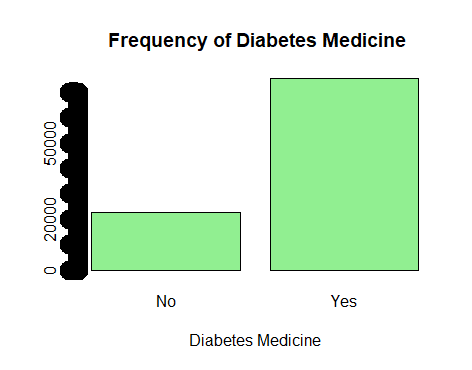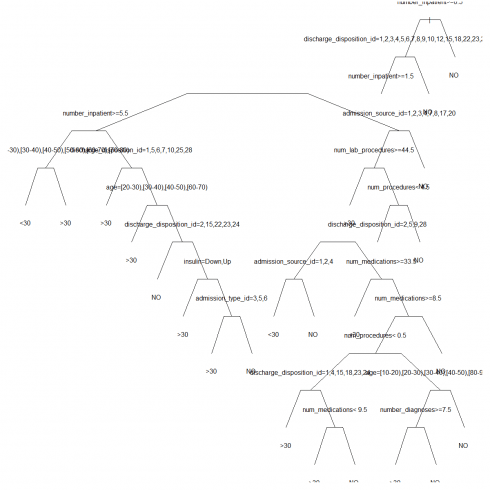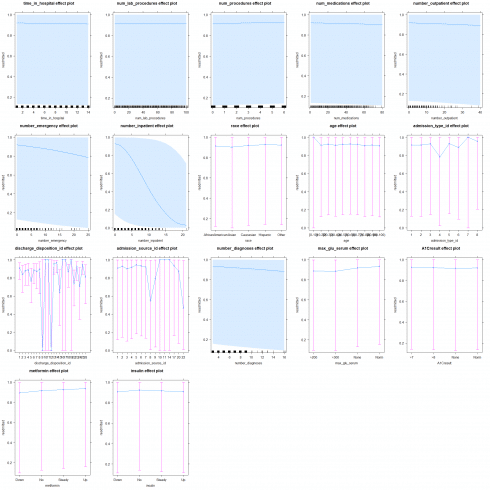# Machine learning: Logistic regression and decision trees for healthcare in R

October 25, 2018
By

(This article was first published on R Programming – DataScience+, and kindly contributed to R-bloggers)

Categories

Tags

One of the sectors with the most demand for machine learning statistics is the healthcare sector and the life science industry. This sector has always been data-driven, looking for evidence about which treatment gives a positive result for the population. In this article you learn how to perform machine learning with logistic regression & decision trees for healthcare and life science industry in R.

## Read packages into R library

First we need to load packages into R library

```library(caret)
library(rpart)
library(tree)
library(skimr)
library(stargazer)
```

### Datacase: Diabetes treatment data

We use this diabetes data that can be downloaded here. After reading the dataset we see at some descriptive statistics with the `skimr` package:

```# Read dataset into R
diabetes1 <- subset(diabetes,select=-c(encounter_id, patient_nbr, examide,citoglipton,weight, payer_code, medical_specialty))
diabetes2 <- diabetes1[diabetes1\$race != "?",]
diabetes2 <- diabetes2[diabetes2\$diag_1 != "?",]
diabetes2 <- diabetes2[diabetes2\$diag_2 != "?",]
diabetes2 <- diabetes2[diabetes2\$diag_3 != "?",]
# binary representation of readmitted within 30 days
# To make factor of levels
diabetes3 <- cbind(diabetes2[c(7:13,17)], lapply(diabetes2[c(1:6,14:16,18:44)],factor))
skim(diabetes3)
Skim summary statistics
n obs: 98053
n variables: 44

-- Variable type:factor --------------------------------------------------------
variable missing complete     n n_unique                                     top_counts ordered
A1Cresult       0    98053 98053        4      Non: 81860, >8: 7631, Nor: 4854, >7: 3708   FALSE
acarbose       0    98053 98053        4            No: 97754, Ste: 286, Up: 10, Dow: 3   FALSE
acetohexamide       0    98053 98053        2                       No: 98052, Ste: 1, NA: 0   FALSE
admission_source_id       0    98053 98053       17          7: 55951, 1: 28356, 17: 6602, 4: 2945   FALSE
admission_type_id       0    98053 98053        8          1: 52178, 3: 18194, 2: 17543, 6: 5135   FALSE
age       0    98053 98053       10 [70: 25306, [60: 21809, [80: 16702, [50: 16697   ....
....
....

-- Variable type:integer -------------------------------------------------------
variable missing complete     n  mean    sd p0 p25 p50 p75 p100     hist
num_lab_procedures       0    98053 98053 43.15 19.71  1  31  44  57  132 ▂▃▇▆▂▁▁▁
num_medications       0    98053 98053 16.12  8.11  1  11  15  20   81 ▅▇▂▁▁▁▁▁
num_procedures       0    98053 98053  1.35  1.71  0   0   1   2    6 ▇▃▂▂▁▁▁▁
number_diagnoses       0    98053 98053  7.51  1.83  3   6   8   9   16 ▁▃▂▇▁▁▁▁
number_emergency       0    98053 98053  0.2   0.94  0   0   0   0   76 ▇▁▁▁▁▁▁▁
number_inpatient       0    98053 98053  0.65  1.27  0   0   0   1   21 ▇▁▁▁▁▁▁▁
number_outpatient       0    98053 98053  0.38  1.28  0   0   0   0   42 ▇▁▁▁▁▁▁▁
time_in_hospital       0    98053 98053  4.42  2.99  1   2   4   6   14 ▇▇▂▃▂▁▁▁
```

The data set is clean with no missing values.

### Visualizations before anaysis

Let us make some plot of the important variables for the analysis:

```# Plots of important data variables for the analysis
plot(diabetes3\$diabetesMed,col ="lightgreen", xlab = "Diabetes Medicine", main= " Frequency of Diabetes Medicine", lwd =20,pch=18)
```

The above coding gives us the following three plots:
Diabetes:Data looks fine!

## Machine learning with logistic regression & decision trees

Now it is time for testing analysis of the dataset:

```# Testing-training data analysis
set.seed(111)
objTrain <-diabetes3[inTrain,]
objTest <- diabetes3[-inTrain,]
0     1
17398  2214
0         1
0.8871099 0.1128901
0     1
69589  8852
0         1
0.8871509 0.1128491
```

Now lets try to predict with three levels of responses:

```#Prediction with three levels of response variable
cfit <- rpart(readmitted ~ time_in_hospital + num_lab_procedures + num_procedures + num_medications + number_outpatient + number_emergency + number_inpatient + race + age + admission_type_id + discharge_disposition_id + admission_source_id + number_diagnoses + max_glu_serum + A1Cresult + metformin + insulin, data = objTrain, method="class", minsplit = 20, minbucket = 5, cp = 0.001)
par(mar=c(1,1,0.25,1))
plot(cfit, branch = 0.4,uniform = TRUE, compress = TRUE)
text(cfit, pretty = 0)
rpart.predict <- predict(cfit, newdata = objTrain, type="class")
tail(rpart.predict)
```

This gives us the following decision tree logistic regression plot with `rpart`:Let us measure the prediction fit of the analysis:

```#Prediction fit of the analysis
cf
#Mean error rate
mean.error.rate.rpart <- 1- cf\$overall
mean.error.rate.rpart
cf  cf
Confusion Matrix and Statistics

Reference
Prediction  30   NO
30  771 2328 1470
NO  1312 4513 8974

Overall Statistics

Accuracy : 0.583
95% CI : (0.576, 0.5899)
No Information Rate : 0.534
P-Value [Acc > NIR] : < 2.2e-16

Kappa : 0.1877
Mcnemar's Test P-Value : < 2.2e-16

Statistics by Class:

Class: 30 Class: NO
Sensitivity             0.05917     0.3361    0.8570
Specificity             0.99351     0.8233    0.3627
Pos Pred Value          0.53689     0.5095    0.6064
Neg Pred Value          0.89245     0.6943    0.6888
Prevalence              0.11289     0.3532    0.5340
Detection Rate          0.00668     0.1187    0.4576
Detection Prevalence    0.01244     0.2330    0.7546
Balanced Accuracy       0.52634     0.5797    0.6098
```
```printcp(cfit)
Classification tree:
rpart(formula = readmitted ~ time_in_hospital + num_lab_procedures +
num_procedures + num_medications + number_outpatient + number_emergency +
number_inpatient + race + age + admission_type_id + discharge_disposition_id +
admission_source_id + number_diagnoses + max_glu_serum +
A1Cresult + metformin + insulin, data = objTrain, method = "class",
minsplit = 20, minbucket = 5, cp = 0.001)

Variables actually used in tree construction:
 num_lab_procedures       num_medications          num_procedures           number_diagnoses         number_inpatient

Root node error: 9140/19612 = 0.46604

n= 19612

CP nsplit rel error  xerror      xstd
1 0.0468271      0   1.00000 1.00000 0.0076433
2 0.0203501      1   0.95317 0.95317 0.0076132
3 0.0051422      2   0.93282 0.93315 0.0075957
4 0.0014442      5   0.91740 0.92998 0.0075927
5 0.0014223     12   0.90613 0.92899 0.0075918
6 0.0012035     13   0.90470 0.92834 0.0075912
7 0.0011488     15   0.90230 0.92812 0.0075909
8 0.0010284     17   0.90000 0.92976 0.0075925
9 0.0010000     22   0.89486 0.92921 0.0075920
```
```par(mar=c(3,3,3,3))
plotcp(cfit,lty = 3, col = 1)
printcp(cfit) ```

The above coding also gives us the following plot for fit of the analysis:The above analysis tells us the three levels are optimal for the decision tree. Now it is time to validate the decision tree with three levels:

```# Validation of the tree
cfit.tree <- tree(readmitted ~ time_in_hospital + num_lab_procedures + num_procedures + num_medications + number_outpatient + number_emergency + number_inpatient + race + age + admission_type_id + discharge_disposition_id + admission_source_id + number_diagnoses + max_glu_serum + A1Cresult + metformin + insulin, data = objTrain, method="class")
cv.cfit.tree <- cv.tree(cfit.tree, FUN = prune.misclass)
cv.cfit.tree
prune.cfit.tree <- prune.misclass(cfit.tree, best = 4)
#plot(prune.cfit.tree)
text(prune.cfit.tree, pretty = 0)
# After pruning
cfit2 = prune(cfit, cp = 0.0014)
par(mar=c(1,1,0.25,1))
plot(cfit2, branch = 0.4,uniform = TRUE, compress = TRUE)
text(cfit2)
```

The above coding gives us the following logistic regression decision tree visualization:Now let us see a prediction test of the model with three levels of decision trees:

```#Prediction on test set
rpart.prune.predict <- predict(cfit2, newdata = objTest,type = "class")
#Mean error rate
mean.error.rate.rpart.prune <- 1- cf.prune\$overall
mean.error.rate.rpart.prune
mean.error.rate.rpart.prune
Accuracy
0.4312923 ```
```cf.prune\$table
cf.prune\$table
Reference
Prediction   30    NO
30  3628  9735  6957
NO   5216 17951 34867```
```# Decision Tree with two class response variable
cfit_bin <- rpart(readmitted ~ time_in_hospital + num_lab_procedures + num_procedures + num_medications + number_outpatient + number_emergency + number_inpatient + race + age + admission_type_id + discharge_disposition_id + admission_source_id + number_diagnoses + max_glu_serum + A1Cresult + metformin + insulin, data = objTrain, method="class", minsplit = 1, minbucket = 1, cp = 0.001)
par(mar=c(2,2,0.25,1))
plot(cfit_bin, branch = 0.4,uniform = TRUE, compress = TRUE)
text(cfit_bin, pretty = 0) ```

The above coding also gives us a visualization a model with two levels of decision trees:Let us make a ROC curve of the predicition level of the model:

```rpart.predict_bin <- predict(cfit_bin, newdata = objTrain,type="prob")
Area under the curve (AUC): 0.591
```

Now let us display the final model in a logistic regression:

```# Display the model wit logistic rergression
logregmod <- glm(readmitted ~ time_in_hospital + num_lab_procedures + num_procedures + num_medications + number_outpatient + number_emergency + number_inpatient + race + age + admission_type_id + discharge_disposition_id + admission_source_id + number_diagnoses + max_glu_serum + A1Cresult + metformin + insulin,
data = objTrain, family = binomial(link= "logit"))
# Display regression models in a table

==============================================
Dependent variable:
---------------------------
----------------------------------------------
time_in_hospital             -0.009
(0.009)
num_lab_procedures           0.0002
(0.001)
num_procedures                0.026
(0.016)
num_medications              -0.003
(0.004)
number_outpatient            -0.008
(0.017)
number_emergency             -0.045*
(0.024)
number_inpatient            -0.288***
(0.016)
...
...
insulinUp                     0.019
(0.091)
Constant                     15.894
(691.210)
----------------------------------------------
Observations                 19,612
Log Likelihood             -6,469.063
Akaike Inf. Crit.          13,094.130
==============================================
Note:              *p<0.1; **p<0.05; ***p<0.01
```

Now let us display the marginal effects of the above logistic model, because they are better for interpretation of the model:

```# Effects & marginal effects
library(effects)
library(mfx)
# effects model
eff <- allEffects(logregmod)
plot(eff, rescale.axis = FALSE)
# Marginal effects model
mfx <-logitmfx(readmitted ~ time_in_hospital + num_lab_procedures + num_procedures + num_medications + number_outpatient + number_emergency + number_inpatient + race + age + admission_type_id + discharge_disposition_id + admission_source_id + number_diagnoses + max_glu_serum + A1Cresult + metformin + insulin,
data = objTrain, atmean = TRUE, robust = FALSE)
mfx
mfx
Call:
logitmfx(formula = readmitted ~ time_in_hospital + num_lab_procedures +
num_procedures + num_medications + number_outpatient + number_emergency +
number_inpatient + race + age + admission_type_id + discharge_disposition_id +
admission_source_id + number_diagnoses + max_glu_serum +
A1Cresult + metformin + insulin, data = objTrain, atmean = TRUE,
robust = FALSE)

Marginal Effects:
dF/dx   Std. Err.       z     P>|z|
time_in_hospital           -7.0364e-04  1.4691e-03 -0.4790  0.631958
num_lab_procedures          1.7745e-05  1.1263e-04  0.1575  0.874814
num_procedures              1.9928e-03  3.8622e-03  0.5160  0.605877
num_medications            -2.0157e-04  4.6671e-04 -0.4319  0.665815
number_outpatient          -5.6791e-04  1.6617e-03 -0.3418  0.732519
number_emergency           -3.4167e-03  6.5285e-03 -0.5234  0.600727
number_inpatient           -2.1719e-02  3.9921e-02 -0.5440  0.586417
raceAsian                  -8.2724e-03  2.6997e-02 -0.3064  0.759287
raceCaucasian               6.6066e-03  1.2992e-02  0.5085  0.611086
raceHispanic                1.4005e-02  2.8637e-02  0.4890  0.624814
raceOther                   1.0805e-02  2.4138e-02  0.4476  0.654423
age[10-20)                 -9.2232e-01  2.9758e-01 -3.0994  0.001939 **
age[20-30)                 -9.3198e-01  6.9720e-01 -1.3368  0.181301
age[30-40)                 -9.4820e-01  1.2347e+00 -0.7679  0.442520
age[40-50)                 -9.7652e-01  1.5383e+00 -0.6348  0.525558
age[50-60)                 -9.9171e-01  1.0409e+00 -0.9528  0.340714
...
...
...
insulinUp                   1.4374e-03  7.2518e-03  0.1982  0.842881
---
Signif. codes:  0 ‘***’ 0.001 ‘**’ 0.01 ‘*’ 0.05 ‘.’ 0.1 ‘ ’ 1
```

The above coding also gives the following effect visualization:### References

Related Post

R-bloggers.com offers daily e-mail updates about R news and tutorials on topics such as: Data science, Big Data, R jobs, visualization (ggplot2, Boxplots, maps, animation), programming (RStudio, Sweave, LaTeX, SQL, Eclipse, git, hadoop, Web Scraping) statistics (regression, PCA, time series, trading) and more...